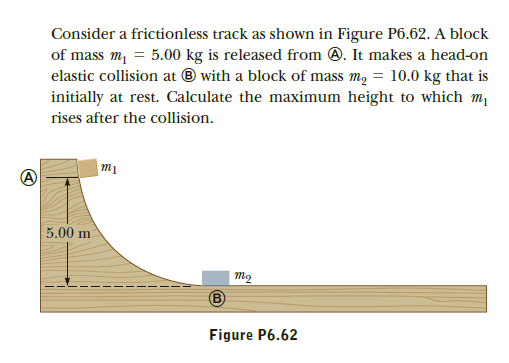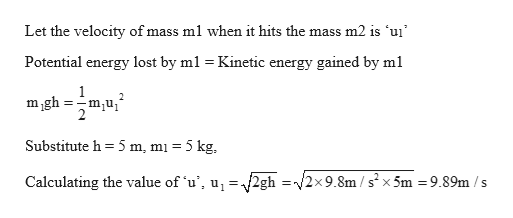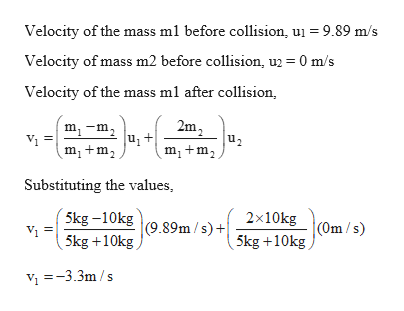# Consider a frictionless track as shown in Figure P6.62. A blockof mass m, = 5.00 kg is released from 0. It makes a head-onelastic collision at ® with a block of mass m, = 10.0 kg that isinitially at rest. Calculate the maximum height to which mrises after the collision.5.00 mтоFigure P6.62

Question
2 viewshelp_outlineImage TranscriptioncloseConsider a frictionless track as shown in Figure P6.62. A block of mass m, = 5.00 kg is released from 0. It makes a head-on elastic collision at ® with a block of mass m, = 10.0 kg that is initially at rest. Calculate the maximum height to which m rises after the collision. 5.00 m то Figure P6.62 fullscreen
check_circle

Step 1

Given:

Mass m1 = 5kg

Mass  m2 = 10 kg

Step 2

Calculating the velocity of mass m1 before it hits the mass m2:help_outlineImage TranscriptioncloseLet the velocity of mass ml when it hits the mass m2 is 'uį' Potential energy lost by ml = Kinetic energy gained by ml mgh = -m,u, Substitute h = 5 m, m1 = 5 kg, Calculating the value of 'u', u, =2gh =/2x9.8m / s²x 5m = 9.89m / s fullscreen
Step 3

Calculating the velocity of the...help_outlineImage TranscriptioncloseVelocity of the mass ml before collision, uj = 9.89 m/s 0 m/s Velocity of mass m2 before collision, u2 = Velocity of the mass ml after collision, m, -m, 2m, u2 m, +m, m,+m, Substituting the values, 5kg –10kg Vi = 5kg +10kg 2x10kg (9.89m / s) + (Om /s) 5kg +10kg Vị =-3.3m /s || fullscreen

### Want to see the full answer?

See Solution

#### Want to see this answer and more?

Solutions are written by subject experts who are available 24/7. Questions are typically answered within 1 hour.*

See Solution
*Response times may vary by subject and question.
Tagged in

### Kinematics